Next: Low-rank approximations Up: Matrix decompositions and latent Previous: Matrix decompositions   Contents   Index

# Term-document matrices and singular value decompositions

The decompositions we have been studying thus far apply to square matrices. However, the matrix we are interested in is the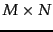term-document matrix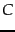where (barring a rare coincidence)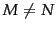; furthermore,is very unlikely to be symmetric. To this end we first describe an extension of the symmetric diagonal decomposition known as the singular value decomposition . We then show in Section 18.3 how this can be used to construct an approximate version of. It is beyond the scope of this book to develop a full treatment of the mathematics underlying singular value decompositions; following the statement of Theorem 18.2 we relate the singular value decomposition to the from Section 18.1.1 . Given, let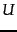be the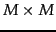matrix whose columns are the orthogonal eigenvectors of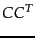, and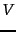be the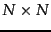matrix whose columns are the orthogonal eigenvectors of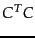. Denote by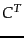the transpose of a matrix.

Theorem. Let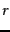be the rank of thematrix. Then, there is a singular-value decomposition ( SVD for short) ofof the form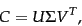(232)

where
1. The eigenvalues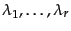ofare the same as the eigenvalues of;
2. For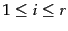, let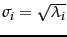, with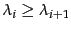. Then thematrix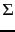is composed by setting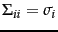for, and zero otherwise.
End theorem.

The values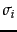are referred to as the singular values of. It is instructive to examine the relationship of Theorem 18.2 to Theorem 18.1.1; we do this rather than derive the general proof of Theorem 18.2, which is beyond the scope of this book.

By multiplying Equation 232 by its transposed version, we have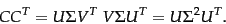(233)

Note now that in Equation 233, the left-hand side is a square symmetric matrix real-valued matrix, and the right-hand side represents its symmetric diagonal decomposition as in Theorem 18.1.1. What does the left-hand siderepresent? It is a square matrix with a row and a column corresponding to each of the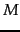terms. The entry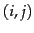in the matrix is a measure of the overlap between the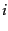th and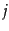th terms, based on their co-occurrence in documents. The precise mathematical meaning depends on the manner in whichis constructed based on term weighting. Consider the case whereis the term-document incidence matrix of page 1.1 , illustrated in Figure 1.1 . Then the entryinis the number of documents in which both termand termoccur.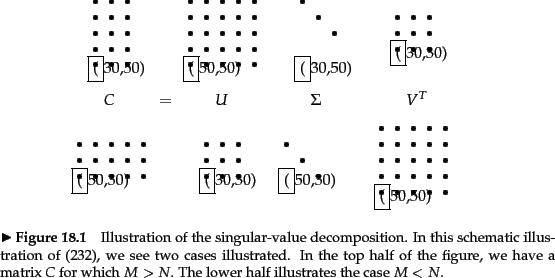When writing down the numerical values of the SVD, it is conventional to representas an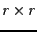matrix with the singular values on the diagonals, since all its entries outside this sub-matrix are zeros. Accordingly, it is conventional to omit the rightmost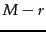columns ofcorresponding to these omitted rows of; likewise the rightmost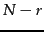columns ofare omitted since they correspond in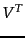to the rows that will be multiplied by thecolumns of zeros in. This written form of the SVD is sometimes known as the reduced SVD or truncated SVD and we will encounter it again in Exercise 18.3 . Henceforth, our numerical examples and exercises will use this reduced form.

Worked example. We now illustrate the singular-value decomposition of a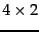matrix of rank 2; the singular values are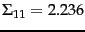and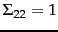.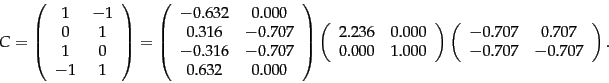(234)

End worked example.

As with the matrix decompositions defined in Section 18.1.1 , the singular value decomposition of a matrix can be computed by a variety of algorithms, many of which have been publicly available software implementations; pointers to these are given in Section 18.5 .

Exercises.

• Let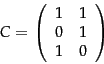(235)

be the term-document incidence matrix for a collection. Compute the co-occurrence matrix. What is the interpretation of the diagonal entries ofwhenis a term-document incidence matrix?

• Verify that the SVD of the matrix in Equation 235 is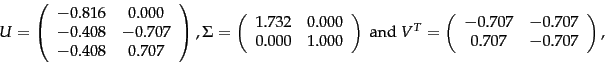(236)

by verifying all of the properties in the statement of Theorem 18.2.

• Suppose thatis a binary term-document incidence matrix. What do the entries ofrepresent?

• Let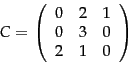(237)

be a term-document matrix whose entries are term frequencies; thus term 1 occurs 2 times in document 2 and once in document 3. Compute; observe that its entries are largest where two terms have their most frequent occurrences together in the same document.Next: Low-rank approximations Up: Matrix decompositions and latent Previous: Matrix decompositions   Contents   Index